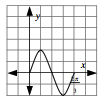### Home > APCALC > Chapter 1 > Lesson 1.4.3 > Problem1-174

1-174.

A focus of this course will be determining maximum and minimum values of a function on a given interval. However, you already have the skills to do this for certain functions. On your paper, sketch $y = 2 \sin(3x)$ for $\frac { 2 \pi } { 3 }$. Determine the points at which the function is a maximum and a minimum.

See graph at right.

$\text{It is a sine curve with amplitude 2 and period }\frac{2\pi}{3}.$

The graph is a sine curve that is vertically stretched by a factor of $2$ and horizontally compressed by a factor of $3$.$\text{Maximum at } \left(\frac{\pi}{6},?\right), \text{ minimum at } (?,-2).$